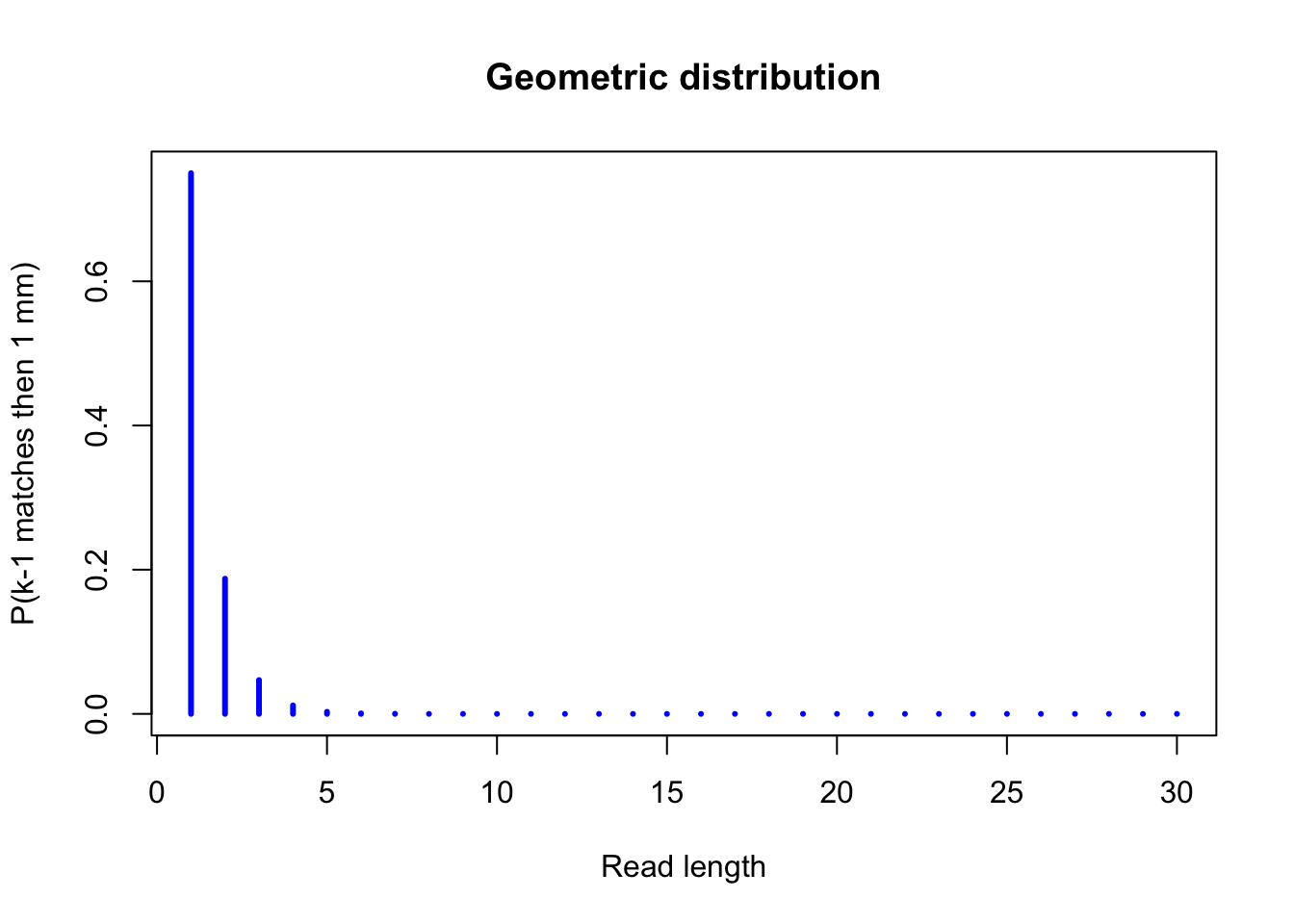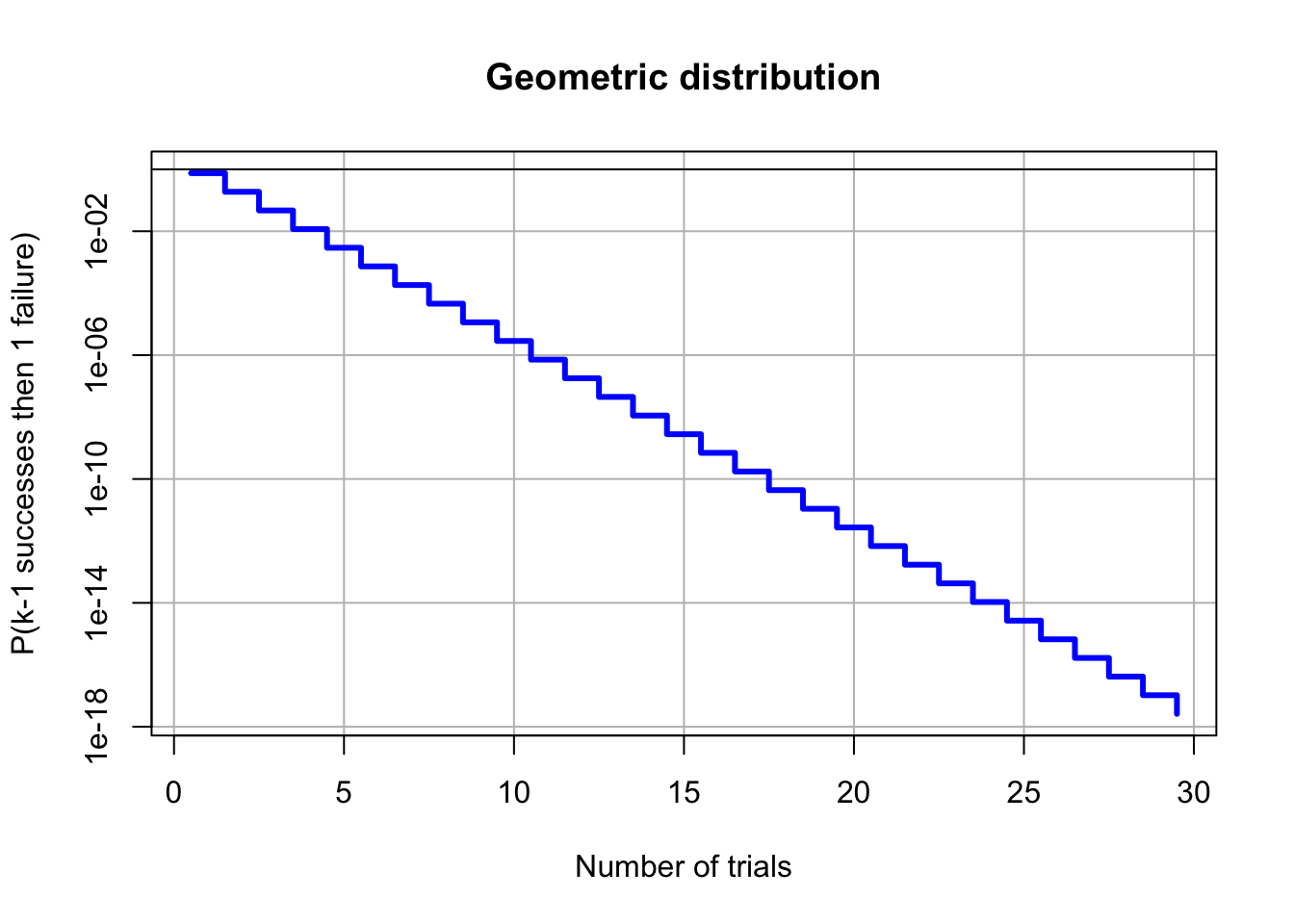Author: Jacques van Helden

## Objectives

In a first time, we will consider the probability of matching one particular read against one particular genomic position. More precisely, if we extract a genomic sequence of length k starting at an arbitrary position of a given chromosome, what would be the probability of this particular k-mer to match this particular read?

For this exercise, we will rely on the simplifying assumption that nucleotides are equiprobable and distributed independently along genomic sequences.

We will progressively approach the solution by resolving successive problems, from the simplest case (perfect match probability) to the actual case of interest (probability of match with at most m mismatches).

## Questions

Compute the probability for the following matching criteria:

1. Perfect match between the read sequence and the oligonucleotide of the length k (k-mer) found at a given position [i:(i+k-1)] of the genome.
2. Single mismatch at the end: k-1 matches followed by one mismatch.
3. Not a single matching residue over the whole length of the read.
4. Exactly one mismatch, which can be at any position of the read.
5. At most one mismatch, which can be at any position of the read.
6. Match of the x=30 first nucleotides, irrespective of what follows.
7. Match of the x=30 first nucleotides, followed by a mismatch (irrespective of what follows).
8. Match of the x=30 first nucleotides, followed by m=k-x=5 mismatches.
9. Exactly x matching nucleotides (exactly m=k-x mismatches), irrespective of their positions.
10. At least x=30 matching nucleotides (at most m=k-x=5 mismatches).
11. For each of these matching types, compute the number of matches expected by chance (e-value) when the mapping is done on the whole genome, for a single read.
12. For each of these, compute the e-value for the whole library.
13. Draw a plot showing the number of expected matches (full-library, genome-wide) as a function of the accepted mismatches.

## Solutions

For each step of thje exercise, we present the R code to perform the computation (shaded rounded boxes) followed by the results and figures.

################################################################
## Define the parameters of the problem
k <- 35     ## Read length
G <- 3e+9   ## Genome size (on a single strand)
p <- 1/4    ## Prior probability to match a residue (assuming equiprobable nucleotides)
N <- 50e6   ## Library size, i.e. number of reads to be mapped

### 1. Perfect match at a given genomic position

If we assume equiprobable nucleotides, the prior probability for a nucleotide of the read to match a genomic nucleotide is $$p = 1/4$$.

Since we also assume that the nucleotides succeed each other independently, we can compute the joined probability of 35 matching nucleotide as the product of the probabilities of nucleotide matches.

$P_1 = p^k$

## Create a vector to store the probabilities
proba <- vector()

## Compute the probability of perfect match, and store it in the "proba" vector
proba["perfect.match"] <- p^k
print(proba)
perfect.match
8.470329e-22 

### 2. Single mismatch at the end: k-1 matches followed by one mismatch.

$P_2 = p^{k-1} (1-p)$

## Compute the probability of observing k-1 matches followed by a single mismatch
proba["last.mm"] <- p^(k-1) *(1-p)
print(proba)
perfect.match       last.mm
8.470329e-22  2.541099e-21 

We can now generalize the case, and compute the same probability for any sequence length ($$x$$) from 1 to 30. The resulting formula corresponds to the geometric distribution.

## Generalize the case: compute the geometric density for any value of x
x <- 1:30
dens.geo <- p^(x-1) * (1-p)
plot(x, dens.geo,
type="h", # Histogram type of line is appropriate for discrete distributions
lwd=3, col="blue",
main="Geometric distribution",
xlab="Read length", ylab="P(k-1 matches then 1 mm)")We can easily ask R to print the Y values on a logarithmic scale, in order to better distinguish the small probabilities.

## Print the same distibution with a logarithmic scale
plot(x-1/2, dens.geo, log="y",
type="s", # Histogram type of line is appropriate for discrete distributions
lwd=3, col="blue",
main="Geometric distribution",
xlab="Number of trials",
ylab="P(k-1 successes then 1 failure)",
panel.first=grid(lty="solid", col="gray")
)
abline(h=1, col="black")### 3. Not a single matching residue over the whole length of the read.

$P_3 = (1-p)^k$

proba["full.mm"] <- (1-p)^k
print(as.data.frame(proba))
                     proba
perfect.match 8.470329e-22
last.mm       2.541099e-21
full.mm       4.237837e-05

### 4. Exactly one mismatch, which can be at any position of the read.

$P_4 = k \cdot p^{k-1} (1-p)$

## Probability to obtain exactly one mismatch, at any position of the k-mer
proba["one.mm"] <- proba["last.mm"] *k
print(as.data.frame(proba))
                     proba
perfect.match 8.470329e-22
last.mm       2.541099e-21
full.mm       4.237837e-05
one.mm        8.893846e-20

### 5. At most one mismatch, which can be at any position of the read.

$P_5 = p^k + k \cdot p^{k-1} (1-p)$

proba["at.most.one.mm"] <- proba["one.mm"] + proba["perfect.match"]
print(as.data.frame(proba))
                      proba
perfect.match  8.470329e-22
last.mm        2.541099e-21
full.mm        4.237837e-05
one.mm         8.893846e-20
at.most.one.mm 8.978549e-20

### 6. Match of the x=30 first nucleotides, irrespective of what follows.

$P_6 = p^x$

x <- 30
proba["x.first.matches"] <- p^x
print(as.data.frame(proba))
                       proba
perfect.match   8.470329e-22
last.mm         2.541099e-21
full.mm         4.237837e-05
one.mm          8.893846e-20
at.most.one.mm  8.978549e-20
x.first.matches 8.673617e-19

### 7. Match of the x=30 first nucleotides, followed by a mismatch (irrespective of what follows).

$P_7 = p^x (1-p)$

x <- 30
proba["x.matches.then.one.mm"] <- p^x * (1-p)
print(as.data.frame(proba))
                             proba
perfect.match         8.470329e-22
last.mm               2.541099e-21
full.mm               4.237837e-05
one.mm                8.893846e-20
at.most.one.mm        8.978549e-20
x.first.matches       8.673617e-19
x.matches.then.one.mm 6.505213e-19

### 8. Match of the x=30 first nucleotides, followed by m=k-x=5 mismatches.

$P_8 = p^x (1-p)^{k-x}$

x <- 30
proba["x.matches.then.all.mm"] <- p^x * (1-p)^(k-x)
print(as.data.frame(proba))
                             proba
perfect.match         8.470329e-22
last.mm               2.541099e-21
full.mm               4.237837e-05
one.mm                8.893846e-20
at.most.one.mm        8.978549e-20
x.first.matches       8.673617e-19
x.matches.then.one.mm 6.505213e-19
x.matches.then.all.mm 2.058290e-19

### 9. Exactly x matching nucleotides (exactly m=k-x mismatches), irrespective of their positions.

$P_9 = C_k^x p^x (1-p)^{k-x}$

x <- 30
proba["x.matches.exactly"] <- choose(n=k, k=x) * p^x * (1-p)^(k-x)
print(as.data.frame(proba))
                             proba
perfect.match         8.470329e-22
last.mm               2.541099e-21
full.mm               4.237837e-05
one.mm                8.893846e-20
at.most.one.mm        8.978549e-20
x.first.matches       8.673617e-19
x.matches.then.one.mm 6.505213e-19
x.matches.then.all.mm 2.058290e-19
x.matches.exactly     6.681868e-14

This is the binomial density !

We can check this by using the R function dbinom()

proba["dbinom"] <- dbinom(x=x, size=k, prob=p)
print(as.data.frame(proba))
                             proba
perfect.match         8.470329e-22
last.mm               2.541099e-21
full.mm               4.237837e-05
one.mm                8.893846e-20
at.most.one.mm        8.978549e-20
x.first.matches       8.673617e-19
x.matches.then.one.mm 6.505213e-19
x.matches.then.all.mm 2.058290e-19
x.matches.exactly     6.681868e-14
dbinom                6.681868e-14

We will now plot the binomial density function for all possible values of $$x$$, from $$0$$ to $$k$$.

x.values <- 0:k
print(x.values)
   0  1  2  3  4  5  6  7  8  9 10 11 12 13 14 15 16 17 18 19 20 21 22
 23 24 25 26 27 28 29 30 31 32 33 34 35
y.values <- dbinom(x=x.values, size=k, prob=p)
print(y.values)
  4.237837e-05 4.944143e-04 2.801681e-03 1.027283e-02 2.739421e-02
 5.661471e-02 9.435785e-02 1.303037e-01 1.520210e-01 1.520210e-01
 1.317515e-01 9.981175e-02 6.654117e-02 3.924223e-02 2.055545e-02
 9.592544e-03 3.996894e-03 1.489039e-03 4.963463e-04 1.480331e-04
 3.947549e-05 9.398927e-06 1.993712e-06 3.756268e-07 6.260447e-08
 9.181989e-09 1.177178e-09 1.307976e-10 1.245691e-11 1.002280e-12
 6.681868e-14 3.592402e-15 1.496834e-16 4.535861e-18 8.893846e-20
 8.470329e-22
plot(x.values,y.values, type="h", col="blue", lwd=2,
xlab="x",
ylab="dbinom(x,k,p)",
main="Binomial density function")
grid()